The ring systems having following characteristics are aromatic.

1. Planar ring containing conjugated $\mathrm{\pi }$ bonds.

2. Complete delocalisation of the $\mathrm{\pi }$-electrons in ring system i.e., each atom in the ring has unhybridised p-orbital, and

3.  Presence of $\left(4\mathrm{n}+2\right)\mathrm{\pi }$-electrons in the ring where n is an integer (n=0,1,2,...) [Huckel rule].

Using this information classify the following compounds as aromatic/non-aromatic.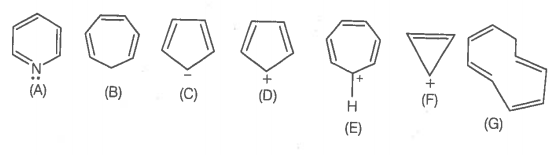Compound Planar Ring Complete delocalization of -electron Huckel rule electron Aromatic or non-aromatic A.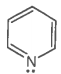Yes Yes $6{\mathrm{\pi e}}^{-}$ Huckel rule obeyed Aromatic B.No No, Incomplete (sp3 hybrid carbon) $6{\mathrm{\pi e}}^{-}$ Non-aromatic C.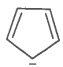Yes Yes $6{\mathrm{\pi e}}^{-}$(4n+2+lone pair e-) Huckel rule verified Aromatic D.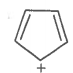Yes No $4{\mathrm{\pi e}}^{-}$ Anti-aromatic E.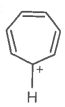Yes Yes Huckel rule obeyed Aromatic F.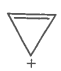Yes Yes $2{\mathrm{\pi e}}^{-}$ Huckel rule verified n=0 Aromatic G.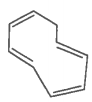Yes No $8{\mathrm{\pi e}}^{-}$ Huckel rule not verified Non-aromatic